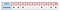# The Markov Chain Model

If you have suffered the Statistics class in college, you probably have heard about the term, the Markov Chain. Markov Chain is essentially a sequence of events in which the probability of each event depends only on the state attained in the previous event. Sounds abstract? It is actually an every powerful statistical model to help us understand how the business is performing. In this article, I will go over the basic concept of Markov Chain and how we can apply the Markov Model to unlock some business insights.

Markov Chain is used to model a series of events. Each sequence usually is composed by various events and the order and the length of the sequence can vary drastically different from sample to sample. This chain of events are usually very hard to describe with deterministic statistics. For example, in a monthly subscription service, a user can choose whether they want to subscribe or not each month. One user’s subscription history may look like something like this:Example of a user’s subscription history. Checks represent subscription, or Active state, and empty boxes represent no subscription, or Disabled state.

In this example, a user have two types of events: subscribed and not subscribed. It is easy to see that out of 10 months, the member is active for 6 months. However, it is hard to describe the fact that the user has switched on and off several times, and here goes the Markov Chain Model.

States of the Markov Chain Model

Let us put this data into a Markov model, and it has two states: Active (A) and Disabled(D). The probability of transition from one state to the other can be described in the following diagram.

Specifically, if a user is at Active State, the probability of transitioning into Disabled State is P(A->D) and the probability of staying at Active is P(A->A). Since only these two options are available, the summation of P(A->D) and P(A->A) should equal to 1.

Calculate the Transition Probabilities

Now let us use the data to calculate these transition probabilities. At each month, the user needs to make a decision of whether he or she wants to move from one state to the other. Let’s transform the subscription sequence into the transition pairs:

In this table, we can observe that out of 5 times the member started with an Active States, this member remained Active 3 times, and switched to Disabled State twice (pair 3->4 and pair 7->8). Therefore, P(A->A) = 3/5 = 60% and P(A->D) = 2/5 = 40%. Similarly, P(D->D) = 2/4 = 50% and P(D->A) = 2/4 = 50%. The transition matrix can be summarized as:

Interpretation and Prediction

So how do we interpret these probabilities in term of the business performance? In this example, P(A->D) is usually interpreted as the churning rate and P(D->A) is often phrased as the reactivation rate. The churning rate is a quantitative metric of how often the users are retaining and often involved in the projection of long-term growth. The reactivation rate is an even more interesting metric because it signifies the efforts that have been put into reviving the churned customers. Continuous monitoring of these two rates will provide a much better picture of user’s subscription behavioral pattern than the simple measure of overall active rate being 60%.

Another way of using the Markov Model is to make predictions. The questions we can answer are:

For a user at the Active State right now, what is the probability of staying active in 1 month and 5 months?

The calculation entails multiplication of the vector of current state ([1, 0]) and the transition matrix:

Therefore, the probability of this member staying alive is 0.6 in one month and 0.56 in five months.

Other Applications of Markov Chain Model

To demonstrate the concept of Markov Chain, we modeled the simplified subscription process with two different states. In the real-life application, the business flow will be much more complicated than that and Markov Chain model can easily adapt to the complexity by adding more states.

Another event series that is commonly modeled by Markov Chain is customer experience events, which encompass a sequence of touch points with the customer, such as the ads, emails and other forms of communications. The transition matrix from the Markov Chain model can be used as a quantitative metric of the Customer Relationship Management (CRM) efficiency and to attribute the credits of each type of touch points.

In some applications, the states of the system are not observable. In the previous example, a member in Disabled state can be soft-churned (intended to come back in a few months) or hard-churned (won’t come back again), and we have no way to obtain the true intention of a customer. In this case, Hidden Markov Model will be of great use to construct a Markov model with unobservable/hidden states.

Assumptions and Limitations of Markov Model

The Markov Chain is very powerful when modeling stochastic processes such as ordering and CRM events. In order to make the correct interpretation, I would like to review some of the assumptions of the Markov Model and the scenarios where this model will fall short.

Assumption 1: The probabilities apply to all participants in the system.

Markov Model assumes everyone in the customer base are the same and any analysis associated with personalization or cohort characterization cannot use this method.

Assumption 2: The transition probabilities are constant over time.

This assumption presumes customer’s first month churning rate is the same as customer’s 10th month churning rate, which in reality is rarely the case.

Assumption 3: The states are independent over time.

This assumption is violated when a customer has been in the Disabled state for a long time and the probability of coming back is very low.